Home | | Finite Element Analysis | Two Dimensional Finite Element Analysis

# Two Dimensional Finite Element Analysis

Three Noded Linear Triangular Element, Four Noded Linear Rectangular Element, Two-Variable 3-Noded Linear Triangular Element, Strain – Stress Relation, 1 Plane Stress Conditions, 2 Plane Strain Conditions, Generalized Coordinates Approach To Nodel Approximations, Isoparametric Elements, Structural Mechanics Applications In 2 Dimensions, Two Dimensional Finite Element Analysis

TWO DIMENSIONAL FINITE ELEMENT ANALYSIS

INTRODUCTION

Two dimensional elements are defined by three or more nodes in a two dimensional

plane (i.e.,  x,  y plane).  The  basic element  useful for two  dimensional analysis is the

triangular element.Ø Plane Stress and Plane Strain

The 2d element is extremely important for the Plane Stress analysis and Plane

Strain analysis.

Plane Stress Analysis:

It is defined to be a state of stress in which the normal stress (s) and shear

stress (t)directed perpendicular to the plane are assumed to be zero.

Plane Strain Analysis:

It is defined to be a state of strain in which the normal to the xy plane and the

shear strain are assumed to be zero.

THREE NODED LINEAR TRIANGULAR ELEMENT

The physical domain considered is geometrically a 2-Dimensional domain, i.e., an area with uniform thickness and the single variable can be one of pressure, temperature, etc. (a scalar quantity, not a vector quantity). An example is the temperature distribution in a plate. At each point there can be only one temperature. We consider such an area meshed with triangular elements. Each triangular element has three nodes, (i.e., one node at each corner). Let us consider one such element with coordinates one such element with coordinates     (x1,y1), (x2,y2), (x3,y3. The single variable (for example, temperature) at these nodes 1, 2 and 3 are u1 , u 2 and u3 , respectively. If so, then the unknown single variable u (temperature) at any non-nodal point x, y in the 2-D domain can be expressed in terms of the known nodal variables (temperatures) u1 , u 2  and u3.

Let us assume that the single variable can be expressed as

U= c1+c2x+c3y

In order to find the three unknowns c1 , c2  and c3 , we apply the boundary conditionsFOUR NODED LINEAR RECTANGULAR ELEMENTLet us assume that the single variable can be expressed as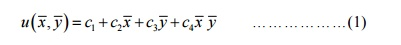This polynomial contains four linearly independent terms and is linear in x and y, with a bilinear term in x and y. The polynomial requires an element with four nodes. There are two possible geometric shapes: a triangle with the fourth node at the centroid of the triangle or a rectangle with nodes at the vertices.

A triangle with a fourth node at the center does not provide a single-valued variation of u at inter-element boundaries, resulting in incompatible variation of u at inter-element boundaries and is therefore not admissible.

The linear rectangular element is a compatible element because on any side, the single variable u varies only linearly and there are two nodes to uniquely define it.

Here we consider an approximation of the form given in eqauation (1) and use a rectangular element with sides a and b. For the sake of convenience we choose a local coordinate system

(x, y  to derive the interpolation functions.In order to find the three unknowns c1 , c2  and c3 , we apply the boundary conditionsTWO-VARIABLE 3-NODED LINEAR TRIANGULAR ELEMENTFigure shows a 2-D two-variable linear triangular element with three nodes and the two dof at each node. The nodes are placed at the corners of the triangle. The two variables (dof) are displacement in x-direction (u) and displacement in y-direction (v). Since each node has two dof, a single element has 6 dof. The nodal displacement vector is given by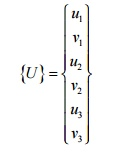We select a linear displacement function for each dof asThe above two algebraic equations can also be written asUsing steps we had developed for the 2-D single-variable linear triangular element, we can write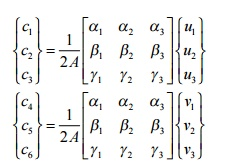and using the interpolation functions we had developed for the 2-D single-variable linear triangular element, we can write{}=[]{a}

strains associated with the two-dimensional element are given by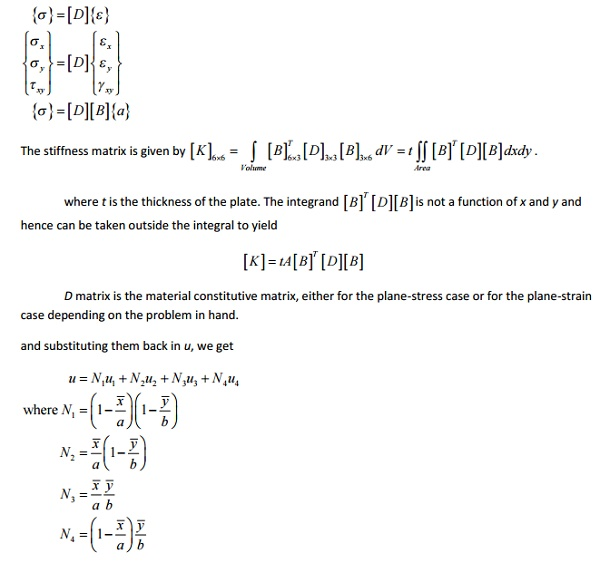STRAIN  STRESS RELATION1 Plane stress conditions

since s z  =0, from equations 1 and 22 Plane strain conditions

s x ,s y  and t xy  are present.

εz  =γxz  =γyz  =0.

s z  is not zero.

since εz  =0, we get from equation 3

z  =(sx  +x )

substituting s in equations 1 and 2It is difficult to represent the curved boundaries by straight edges element a large number of element may be used to obtain reasonable resembalance between original body and the assemblage

Two-Dimensional Problems

Review of the Basic Theory

In general, the stresses and strains in a structure consist of six components:

sx , sy , sz , txy , tyz , tzx for stresses,

ex , e y , ez , g,xy, g,yz , g,zx, for strains.Under contain conditions, the state of stresses and strains can be simplified. A general 3-D structure analysis can, therefore, be reduced to a 2-D analysis.Plane strain:

A long structure with a uniform cross section and transverse loading along its length (z-direction).Stress-Strain-Temperature (Constitutive) Relations

For elastic and isotropic materials, we have,where  e0  is the initial strain  E the Young’s modulus, n  the Poisson’s ratio and G the shear modulus.  Note that,which means that there are only two independent materials constants for homogeneous and isotropic materials.

We can also express stresses in terms of strains by solving the above equation,

The above relations are valid for plane stress case. For plane strain case, we need to replace the material constants in the above equations in the following fashion,

For example, the stress is related to strain by

where a is the coefficient of thermal expansion, T the change of temperature. Note that if the structure is free to deform under thermal loading, there will be no (elastic) stresses in the structure.

GENERALIZED COORDINATES APPROACH TO NODEL APPROXIMATIONSThe boundary S of the body can be divided into two parts, Su and St. The boundary conditions (BCs) are described as, in which tx and ty are traction forces (stresses on the boundary) and the barred quantities are those with known values.

In FEM, all types of loads (distributed surface loads, body forces, concentrated forces and moments, etc.) are converted to point forces acting at the nodes.

Exact Elasticity Solution

The exact solution (displacements, strains and stresses) of a given problem must satisfy the equilibrium equations, the given boundary conditions and compatibility conditions (structures should deform in a continuous manner, no cracks and overlaps in the obtained displacement field)

ISOPARAMETRIC ELEMENTS

In one dimensional problem, each node is allowed to move only in ±x direction. But in two dimensional problem, each node is permitted to move in the two directions i.e., x and y.The element connectivity table for the above domain is explained as table.

Element (e) Nodes

(1)     123

(2)     234

(3)     435

(4)     536

(5)     637

(6)     738

(7)     839

(8)     931

Ø Constant Strain Triangular (CST) Element

A three noded triangular element is known as constant strain triangular (CST)

element. It has six unknown displacement degrees of freedom (u1v1, u2v2, u3v3).Ø Shape function for the CST element Shape function N1 = (p1 + q1x + r1y) / 2A

Shape function N2 = (p2 + q2x + r2y) / 2A Shape function N3 = (p3 + q3x + r3y) / 2A

Ø Displacement function for the CST elementStrain  Displacement matrix [B] for CST elementWhere, q1 = y2 –y3       r1      = x3  –x2

q2 = y3 –y1          r2 = x1 –x3

q3 = y1 –y2          r3 = x2 –x1

Stress  Strain relationship matrix (or) Constitutive matrix [D] for two dimensional elementØ Stress  Strain relationship matrix for two dimensional plane stress problems

The normal stress sand shear stresses tt are zero.Ø Stress  Strain relationship matrix for two dimensional plane strain problems

Normal strain ez and shear strains exz, eyz are zero.

Ø Stiffness matrix equation for two dimensional element (CST element)

Stiffness matrix [k] = [B]T [D] [B] A tFor plane strain problems,

Ø Temperature Effects

Distribution of the change in temperature (ΔT) is known as strain. Due to the

change in temperature can be considered as an initial strain e0.

σ=D(Bu- e0)

Ø Galerkin Approach

Stiffness matrix [K]e = [B]T [D][B] A t.

Force Vector {F}e = [K]e {u}

Ø Linear Strain Triangular (LST) element

A  six  noded  triangular  element  is  known  as  Linear  Strain  Triangular  (LST)

element. It has twelve unknown displacement degrees of freedom. The displacement functions of the element are quadratic instead of linear as in the CST.Ø Problem (I set)

1. Determine the shape functions N1, N2 and N3 at the interior point P for the triangular element for the given figure.The two dimensional propped beam shown in figure. It is divided into two CST elements. Determine the nodal displacement and element stresses using plane stress conditions. Body force is neglected in comparison with the external forces.

Take, Thickness (t) = 10mm,

Young’smodulus(E)=2x105 N/mm2,

Poisson’sratio(v)=0.25.3. A thin plate is subjected to surface traction as in figure. Calculate the global stiffness matrix.Ø Scalar variable problems

In structural problems, displacement at each nodal point is obtained. By using

these displacement solutions, stresses and strains are calculated for each element. In structural problems, the unknowns (displacements) are represented by the components of vector field. For example, in a two dimensional plate, the unknown quantity is the vector field u(x, y), where u is a (2x1) displacement vector.

3.STRUCTURAL8 MECHANICS APPLICATI ONS IN 2 DIMENSION

Elasticity equations are used for solving structural mechanics pr oblems. These equations must be satisfied if an exact solution to a structural mechanics pr oblem is to be obtained. Thest are four basic sets of elasticity equations they are

·        Strain displacement relationship equations

·        Stress strain relationshi p equations

·        Equilibrium equations

·        Compatibility equations

TRUSS ELEMENT

A truss element is defined as a deformable, two-force member that is subjected to loads in the axial direction. T he loads can be tensile or compressive. The only degree of freedom for a one-dimensional truss (bar) element is axial (horizontal) displa cement at each node.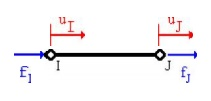Assumptions- Diformensional the One Truss Ele ment

Prismatic Member

The truss element is asssumed to have a constant cross-section, i.e., it is a prismatic member. If a truss structure is stepped, then it must be divided up into sectio ns of constant cross-section in order to obtain an exact solution as shown below.If a truss structure is tapered, then it can be approximated by using maany small truss elements, each having the sa me cross-section as the middle of the tapere d length it is approximating. The more sec tions that are used to approximate a tapered truss, the more accurate the solution will be.Weightless Member

The weight (W) of the truss is neglected since it is assumed to be much less than the total resultant forces (F) actin g on the truss. If the weight of the truss is not n eglected, then its effects must be represented as vertical forces acting at the nodes. But sinc e truss element is defined as two-force member it cannot have any vertical (shear) force, th us the member weight has to be neglected. If shear forces exist, then a beam element must be used to model the structure.

Nodal Forces

For one-dimensional truss element, forces (loads) can only be applied at the nodes of the element, but not between the nodes. This is consistent with the FEM eq uations which relate nodal forces to nodal displacements through the stiffness matrix.

For one-dimensional truss element, forces (loads) can only be applied at the centroid of the element cross-sectional area.

BucklingEffect not Considered

A bar element can be subjected to either tensile or compressive forces. Tensile forces can be applied to a bar of any cross-sectional area or member length, and failure is associated with sudden fracture or general yielding. When compressive forces are applied to a member, it can either fail due to crushing or buckling. Buckling is present when the member bends and laterally deflects as shown on the right figure below.Buckling is not accoun ted for in the formulation of the truss element. Members that do not buckle are classified a s short columns and members that buckles are classified as long columns. The structural response of a short column can be predicte d with a truss element.

To determine if buckling will occur the reader should refer to a mechanics of material textbook. We will now introduce a simple geometric guideline t o determine if buckling might occur. If the ratio between the member length and the least di mension of the cross-section is equal or less th an 10, the member is considered a short colum n and buckling will not occur, i.e.,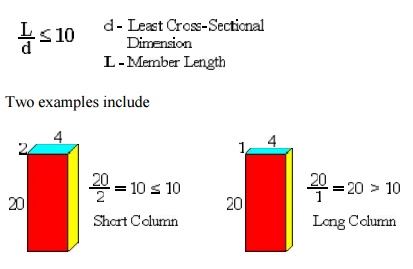Two examples include

In the second case if a bar ele ment is subjected to a compressive force, the element will not predict the buckling response . One should note that the above geometric ru le is a simple guideline, however, in reality buckling depends not only on the member length and cross-sectional area, but material pro perties and support conditions.

Isotropic Ma terial

A truss element has the same mechanical and physical properties in all directtions, i.e., they are independent of direction. F or instance, cutting out three tensile test specimens, one in the x-direction, one in the y-dire ction and the other oriented 45 degrees in the x-plane, a tension test on each specimen, will result in the same mechanical values for the modulus of elasticity (E), yield strengthy and ultimate strengthu. Most metals are considered isotropic. In contrast fibrous materials, such as wood, typically have properties that are directionally dependant and ar e generally considered anisotropic (not isotropic ).

The loads that are applied to t he truss element are assumed to be static and n ot to vary over the time period being considerred. This assumption is only valid if the rate of change of the force is much less than the applied force (F >> dF/dt), i.e., the loads are app lied slowly. If the loads vary significantly, (i f the variation in load is not much less than the applied force) then the problem must be considered as dynamic.

Poisson'sEffect n ot Considered

Poisson's ratio is a material pa rameter. Poisson's effect is when a uniform cr oss-section bar is subject to a tensile load, and the axial stretching is accompanied by a con traction in the lateral dimension. For one-dim ensional truss element., this effect is neglected for simplicity, i.e., v = 0.

Cross Sectio n Remains Plane

For one-dimensional element, although the force(s) are acting on only the c entroid of the truss (bar) element, it is assu med that it has a uniform effect to the plane. Thus the cross section will move uniformly and remain plane and normal to the axial axis before and after loading.

Homogenous M aterial

A truss element has the sam e material composition throughout and therefore the same mechanical properties at every position in the material. Therefore, the modulus of elasticity E is constant throughout the tr uss element. A member in which the material properties varies from one point to the next in the member is called inhomogenous (non-hom ogenous). If a truss is composed of different types of materials, then it must be divide up int o elements that are each of a single homogene ous material, otherwise the solution will not be exact.

The left figure shows a compo site bar composed of brass and aluminum. This structure can be divided into two elements as shown on the right, one element for the brass with E1 = 15 x 106 psi and one for the alumin um with E2 = 10 x 106 psi.TRUSS ELEMENT (OR SPAR ELEMENT OR LINK ELEMENT)

Differentiate between a truss and a frame.

Truss

Loads act only at the joints.

Truss members undergo only a xial deformation (along the length of the member).

Frame

Loads can be applied at the joints and/or in-between the joints

Frame members can undergo axial and bending deformations (translations as well as rotations).

A grid is a structure on which loads applied perpendicular to the plane of the structure, as opposed to a plan e frame, where loads are applied in the plane of the structure.

1 Derivation of stiffness matrix and finite element equation for a truss element.

There are two joints for an arbitrarily inclined single truss element (at an angle q , positive counter-clockwise from +ve x- axis). For each joint i, there are two degrees of freedom, i.e.,

a joint can have horizontal displacement u(i) and vertical displacement v(i) . Hence, for a single truss element, there are 4 degrees of freedom. The nodal displacement degrees of freedom and the nodal force de grees of freedom are shown in the following figgure.Note that the deformations occurring in the truss members are so small that they are only axial. The axial displacement of the truss can be resolved along horizontal x-axis and vertical y-axis. But in our derivation, let us resolve the horizontal and vertical displacements (in xy-axes) of a joint along and perpendicular to the truss member (in x’y-axes). Refer to the Figure in the next page. Note ui sinq component acting towards negative  y -direction and all other components acting towards in +ve x - and  y -directions.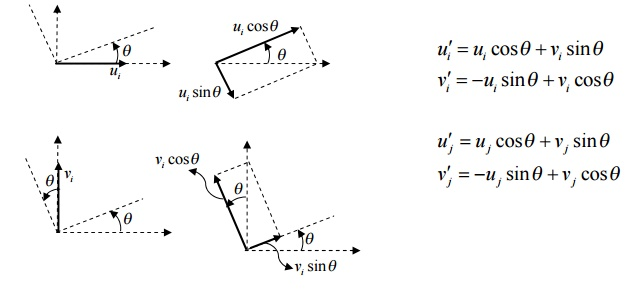The above equations can be written in the matrix form as follows{u¢}=[]{u}

where [T]is the transformation matrix It is important to note that the displacements v ¢(i) and v(i) are both zero since there can be no displacements perpendicular to the length of the member. Also [T ]-1 =[T ]T

Similarly, we resolve forces along the length of the member (positive x direction) and perpendicular to the length of the member (positive y direction){F '}=[]{}

where [T]is the transformation matrix

The arbitrarily inclined truss member can be thought of as a simple bar element oriented at the same angle q . Hence, we can write the finite element equation for this inclined bar element (in x¢ycoordinate system) asSubstituting {F ¢}and {u¢}from the previous equations, we can write

[]{}=[k¢][]{u}

Pre-multiplying the above equation by [T]-1 ,

[]-1 []{F}=[]-1[k¢][T]{u}

But [T ]-1 [T]=1 and the above equation can be written as

{}=[k]{u} where [k] [=T]-1 [k¢][T]

Carrying out the matrix multiplication for [k , we obtainwhere c =cos2 q and s =sin2 q .

Computation of strain and stress in the truss element

The change in length of the truss member is equal to the change in axial displacement of the truss member in the x¢yco-ordinate systemProblem

The two-element truss is subjected to external loading as shown in figure. Using the same node and element numbering as shown in figure, determine the displacement components at node 3, the reaction components at nodes 1 and 2, and the element displacement, stresses and forces. The elements have modulus of elasticity E1 = E2 = 10×106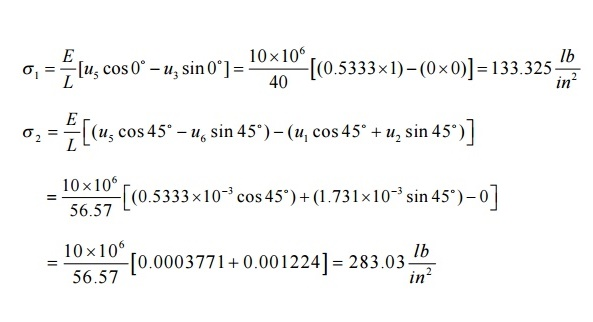PROBLEM

To illustrate how we can combine spring and bar element in one structure, we can solve the two-bar truss supported by a spring as shown below. Both bars have E = 210 GPa and A = 5.0 x10-4 m2. Bar one has a length of 5 m and bar two a length of 10 m. the spring stiffness is k = 2000 kN/m.STEP 4: Applying Boundary Conditions:

Since nodes 1, 2, and 3 are fixed, we have u2 = v2 = 0; u3 = v3 = 0; u4 = v4 = 0;

F1x = 0 and F1y = -25 kNCheck whether there are as many unknowns as knowns.

STEP 5: SOLVING THE EQUATIONS:

Reduced matrix:On solving,

u1=-1.724 x 10-3 m                                              v1=-3.4482 x 10-3 m

Find the reactions at supports by substituting the known nodal values

F2x = -18.104 kN                                       F2y = 18.1041 kN

F3x = 18.102 kN                                        F3y = 0

F4x = 0                                             F4y = 6.89 kN

STEP 6: Post Processing

Stress in element 1:PROBLEM

A circular concrete beam structure is loaded as shown. Find the deflection of points at 8”,16”, and the end of the beam. E = 4 x 106 psi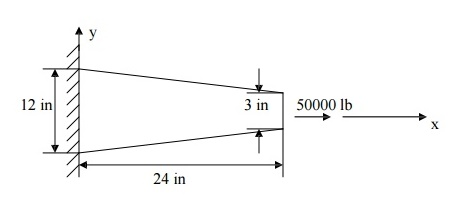Solution

The beam structure looks very different from a spring. However, its behavior is very similar. Deflection occurs along the x-axis only. The only significant difference between the beam and a spring is that the beam has a variable cross-sectional area. An exact solution can be found if the beam is divided into an infinite number of elements, then, each element can be considered as a constant cross-section spring element, obeying the relation F = ku, where k is the stiffness constant of a beam element and is given by k = AE/L.

In order to keep size of the matrices small (for hand- calculations), let us divide the beam into only three elements. For engineering accuracy, the answer obtained will be in an acceptable range. If needed, accuracy can be improved by increasing the number of elements.

As mentioned earlier in this chapter, spring, truss, and beam elements are line-elements and the shape of the cross section of an element is irrelevant. Only the cross-sectional area is needed (also, moment of inertia for a beam element undergoing a bending load need to be defined). The beam elements and their computer models are shown

Here, the question of which cross-sectional area to be used for each beam section arises. A good approximation would be to take the diameter of the mid-section and use that to approximate the area of the element.Cross-sectional area

The average diameters are: d1 = 10.5 in., d2 = 7.5 in., d3 = 4.5. (diameters are taken at the mid sections and the values are found from the height and length ratio of the triangles shown in figure 2.10), which is given as

12/L = 3/(L-24),    L = 32

Average areas are:

A1 = 86.59 in2     A2 = 56.25 in2              A3 = 15.9 in2Stiffness

k1 = A1 E/L1 = (86.59)(4 × 106/8) = 4.3295 ×10 7 lb./in., similarly,

k2 = A2 E/L2 = 2.8125 ×10 7 lb./in.

k3 = A3 E/L3 = 7.95 ×10 6 lb./in.

Element Stiffness EquationsThe deflections u2, u3, and u4 are only the approximate values, which can be improved by dividing the beam into more elements. As the number of elements increases, the accuracy will improve.

Study Material, Lecturing Notes, Assignment, Reference, Wiki description explanation, brief detail
Mechanical : Finite Element Analysis : Two Dimensional Finite Element Analysis : Two Dimensional Finite Element Analysis |

Related Topics